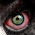## Thursday, December 08, 2011

### Simple heap implementation in C#

I recently needed a simple priority queue in C# and was surprised to find that there is neither anything called "priority queue" nor "min heap" or "max heap" in .NET (or at least I did not find it when I looked). Here is a textbook-like implementation I came up with:

```class MinHeap<T> where T : IComparable
{
private List<T> data = new List<T>();

public void Insert(T o)
{
data.Add(o);

int i = data.Count - 1;
while (i > 0)
{
int j = (i + 1) / 2 - 1;

// Check if the invariant holds for the element in data[i]
T v = data[j];
if (v.CompareTo(data[i]) < 0 || v.CompareTo(data[i]) == 0)
{
break;
}

// Swap the elements
T tmp = data[i];
data[i] = data[j];
data[j] = tmp;

i = j;
}
}

public T ExtractMin()
{
if (data.Count < 0)
{
throw new ArgumentOutOfRangeException();
}

T min = data;
data = data[data.Count - 1];
data.RemoveAt(data.Count - 1);
this.MinHeapify(0);
return min;
}

public T Peek()
{
return data;
}

public int Count
{
get { return data.Count; }
}

private void MinHeapify(int i)
{
int smallest;
int l = 2 * (i + 1) - 1;
int r = 2 * (i + 1) - 1 + 1;

if (l < data.Count && (data[l].CompareTo(data[i]) < 0))
{
smallest = l;
}
else
{
smallest = i;
}

if (r < data.Count && (data[r].CompareTo(data[smallest]) < 0))
{
smallest = r;
}

if (smallest != i)
{
T tmp = data[i];
data[i] = data[smallest];
data[smallest] = tmp;
this.MinHeapify(smallest);
}
}
}
```

#### 15 comments:

1.thanks for this. It helped me

2.Thanks, this is very helpful

3.I have a question though, shouldn't the indexing start from 1 rather than 0?

4.It's a while ago now, so I don't remember the details off the top of my head. Probably the book used 1-indexing (I think I was looking in Norvig's "Artificial Intelligence: A Modern Approach"), and I chose to do it all with 0-indexing because that's more common in C#. I hope I got it right in all the places... Is there a specific line that looks wrong? Thanks!

5.Haven't tested it but I think the problem is when you want to find the children of the root you do 2*0=0 and 2*0+1=1 instead of getting 1 and 2. You don't have such problems when the indexing starts from 1. I hope that makes sense, English is not my first language.

6.You're completely right, there was something very strange there... I have therefore now updated the code to use (i + 1) / 2 - 1 rather than i / 2 . Have also updated the Insert method to be like the pseudocode at http://en.wikibooks.org/wiki/Data_Structures/Min_and_Max_Heaps .

However, for some reason I think it worked before as well (I just did some fuzz testing), I just haven't time currently to figure out why ;-)

7.Here's what I used to fuzz test:

class Program
{
static void Main(string[] args)
{
Random rnd = new Random();

for (int i1 = 0; i1 < 10000; i1++)
{
int n = 1000;
var a = new int[n];

MinHeap h2 = new MinHeap();
for (int i = 0; i < n; i++)
{
a[i] = rnd.Next();
h2.Insert(a[i]);
}

Array.Sort(a);

for (int i = 0; i < n; i++)
{
if (a[i] != h2.Peek())
{
Console.WriteLine("Something went wrong!");
return;
}
h2.ExtractMin();
}
}
}
}

8.Creating a heap is justified only if inserting an element into the heap takes O(log N). In the algorithm, the time taken is O(N) defeats the purpose of creating a heap!

1.What makes you think it is not O(log N)?

2.It is ok as long as the List.Add operation does it in O(1) time. This happens when number of items is lesser than the capacity of the list. When the number of items is greater than the capacity, the insert takes O(N) time. Prefer using a tree or a raw array instead of basing it on top of a List.

3.Just realized that the Capacity of a List can be adjusted at will, anytime and not just at the time of constructor. I guess it is fine.

4.You are right, the implementation of the List collection is a dependency. While I am not sure, I think it grows by a percentage whenever it gets full, so in practice almost all inserts are constant time.

9.Allan, posted an article for MinHeap implementation with a reference to this article. http://vijayt.com/Post/MinHeap-implementation-for-NET

1.Thanks for the reference :-)

10.Awesome! This helped a lot :)